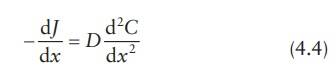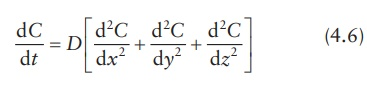# Principles of diffusion

| Home | | Pharmaceutical Drugs and Dosage | | Pharmaceutical Industrial Management |

## Chapter: Pharmaceutical Drugs and Dosage: Biopharmaceutical considerations

Passive diffusion leads to change in concentration in a region over a period of time and space.

Principles of diffusion

Passive diffusion leads to change in concentration in a region over a period of time and space. Fick’s laws of diffusion quantitate the amount of solute diffusing per unit time and area as a function of concentration gradient of solute in the direction of diffusion. These laws also relate the changes in solute concentration in a given region over time to the change in concentra-tion gradient of the solute in that region.

### Fick’s first law

Fick’s law of diffusion postulates that the diffusing molecules go from regions of high concentration to regions of low concentration. The rate of diffusion, the amount of material (M) flowing through a unit cross-section (S) of a barrier in unit time (t) is defined as the flux (J). Flux is related to the concentration gradient (dC = C1C2) between the donor region at a higher concentration (C1) and the receiving region at a lower concentration (C2) per unit distance (x) by the following expression:where:

J is the flux, in g/(cm2s)

S is the cross-section of the barrier, in cm2

dM /dt = rate of diffusion, in g/s (M = mass in g; t = time in s)

The flux is proportional to the concentration gradient, dC/dx:where:

D is the diffusion coefficient of a penetrant, in cm2/s

C is the concentration, in g/cm3 or g/mL

x is the distance perpendicular to the surface of the barrier, in cm

Thus,The negative sign in this equation signifies that diffusion occurs in a direc-tion of decreasing concentration. Thus, the flux is always a positive quantity. Although the diffusion coefficient, D, or diffusivity, as it is often called, appears to be a proportionality constant, it does not remain constant. It is affected by changes in concentration, temperature, pressure, solvent proper-ties, molecular weight, and chemical nature of the diffusant. For example, the larger the molecular weight, the lower the diffusion coefficient.

### Fick’s second law

Fick’s second law predicts changes in solute concentration over time caused by diffusion. It states that the change in concentration with time in a par-ticular region is proportional to the change in the concentration gradient at that region in the system. Concentration of solute or diffusant, C, in the volume of the region, x, changes as a result of the net flow of diffusing molecules in or out of the region. This change in concentration with time, t (i.e., dC/dt), is proportional to the change in the flux of diffusing mol-ecules, J, per unit distance, x (i.e., dJ/dx).Differentiating the equation of flux, J, as per Fick’s first law of diffusion ( J = −D × d C /dx), with respect to x, we obtain:Therefore, concentration and flux are often written as C(x, t) and J(x, t), respectively, to emphasize that these parameters are functions of both dis-tance, x, and time, t. Substituting dC/dt for dJdx, Ficks’s second law of diffusion can be expressed as:This equation represents diffusion only in one direction. To express con-centration changes of diffusant in three dimensions, Fick’s second law of diffusion is written as:Contact Us, Privacy Policy, Terms and Compliant, DMCA Policy and Compliant

TH 2019 - 2022 pharmacy180.com; Developed by Therithal info.